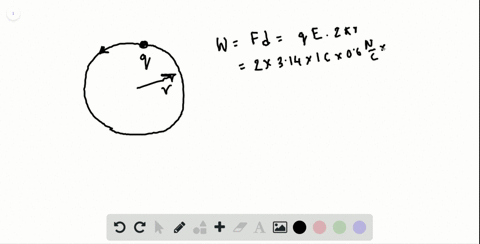🎉 The Study-to-Win Winning Ticket number has been announced! Go to your Tickets dashboard to see if you won! 🎉View Winning Ticket### A wire loop has a radius of 10 $\mathrm{cm} .$ A …

04:04Johns Hopkins University
Problem 48

# You are fixing a transformer for a toy truck that uses an $8.0-\mathrm{V}$ emf to run it. The primary coil of the transformer is broken; the secondary coil has 30 turns. The primary coil is connected to a $120-\mathrm{V}$ wall outlet. (a) How many turns should you have in the primary coil? (b) If you then connect this primary coil to a $240-\mathrm{V}$ source, what emf would be across the secondary coil?

## Discussion

You must be signed in to discuss.

## Video Transcript

in this question. We are trying to fix a transformer for a toy truck, and it looks like the toy truck uses eight volts of, um, F to run, and the primary coil of the transformer is broken and the secondary coil has 30 turns. So let's organize our information. We know that the toy truck will use and the IMF of eight. Oh, and this will be my secondary IMF because it is not the source voltage. It tells us that the primary coil is broken, so we don't know how many turns there are in the primary coil. But we do know that the secondary coil has 30 turns so insipid as this 30 and we do also know the primary voltage source, which is 120 bolts. According to the question. Now, part a asks us, How many turns would you have in the primary coil on DSO? In order to figure this out, we set up our ratio. We know that because the primary and the secondary coils in an ideal transformer should have the same change in magnetic flux over time. And so, by rearranging Faraday's Law of induction, we can also set equality between these two ratios, which is e m f of the primary coil to the number of turns in the primary coil equal to the e m f of the secondary coil, um, time are divided by the number of turns in the secondary coil. So once we have this equality set up, the rest is simply algebra solving for and Seppi we have and some best times uh Yemma Primary divided by the MF secondary and plugging in the numbers. We have 30 times 120 divided by eight and it turns out the primary coil has 450 turns. And this is part A parties asking you if you now connect the primary coil to a 240 voltage source volt source. Ah, what e. M f would be across the secondary coil. So you're going to use, um, this new piece of information, which is that meat? Um, apps. API is now 240 volts, and you just found that the primary coil has up 450 turns. So you're going to use the same ratio equation and you will solve for the secondary yet math rearranging the equation, we get this and then plugging in all the numbers. And so to get 16 volts, that's how yourself this question.